Part 1 | IFT World
IFT Notes for Level I CFA® Program
IFT Notes for Level I CFA® Program

# Part 1

## 1. Introduction

This learning module covers:

• The exercise value, moneyness, and time value of an option
• How arbitrage and replication concepts can be applied to options
• Factors affecting the value of an option

## 2. Option Value relative to the Underlying Spot Price

Options have a non-linear or asymmetric payoff. Their value depends on whether the spot price crosses an exercise threshold at maturity. To gauge an option’s value before maturity, investors frequently rely on three measures – exercise value, moneyness, and time value.

Recall from an earlier reading that American options can be exercised at any time, while European options can be exercised only at maturity. This reading will only focus on European options.

## 3. Option Exercise Value

At expiration, the value of a call and put option can be calculated as:
CT=max (0,ST – X )
PT=max (0,X – ST)
At any time before expiration (t < T), an option’s value is made up of its exercise value and its time value. The exercise value is the option’s value if it could be exercised immediately, whereas the time value captures the possibility that the passage of time and the volatility of the underlying price will increase the profitability of exercise at maturity.
Calculating an Option’s Exercise Value: At any time before expiration (t < T), we can calculate an option’s exercise value by comparing the underlying spot price (St) with the present value of the exercise price (X).
Call Option Exercise Value=max (0,St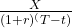Put Option Exercise Value=max [0,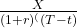– St]

Example: Put Option Exercise Value

(This is based on Example 1 from the curriculum.)

Consider a 1-year put option with an exercise price of EUR 1,000, an initial price of EUR 990, and a risk-free rate of 1%. What is the exercise value of the option in six months if the spot price equals EUR 950?

Solution:

Put Option Exercise Value=max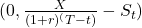Put Option Exercise Value=max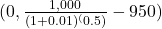= max⁡(0,45.04) = 45.04

Similarity with Forwards:

If we assume an exercise price X, equal to the forward price F0(T), the exercise value of a call option is the same as the value of a long forward commitment: St – PV(F0(T)), provided that the spot price is greater than the present value of X.

Note that this comparison ignores the upfront call premium paid by the option buyer.

## 4. Option Moneyness

An option’s moneyness expresses the relationship between the underlying price and the exercise price. It refers to whether an option would result in a profit or loss if exercised immediately.

• If immediate exercise of the option would result in a positive payoff, then the option is in the money.
• If immediate exercise would result in loss, then the option is out of the money.
• If immediate exercise would result in neither a gain nor a loss, then the option is at the money.

The table below shows the moneyness of an option for various relative values of S and X.

 Call Option Put Option In the money S > X S < X At the money S = X S = X Out of the money S < X S > X

Moneyness is used to compare options on the same underlying but with different exercise prices and/or times to maturity.

Also, the degree to which an option is in or out of the money affects the sensitivity of its price to changes in the price of the underlying.

• Deep-in-the-money options, are highly likely to be exercised, and are therefore highly sensitive to changes in the price of the underlying.
• Whereas, deep-out-of-the money options, are very unlikely to be exercised, and are therefore far less sensitive to changes in the price of the underlying.

Example: Put Option Moneyness

(This is Example 2 from the curriculum.)

Recall in Example 1 that at time t, a put option with six months remaining to maturity had an exercise price (X) of EUR 1,000 and an underlying spot price (St) of EUR 950. Describe the moneyness of the put option at time t.

Solution:

Given that the underlying spot price is below the exercise price, the put option is in the money by EUR 50.

## 5. Option Time Value

Prior to expiration an option also has time value in addition to exercise value. The time value of an option is the amount by which the option premium exceeds the exercise value.

The longer the time to expiration the higher the time value.

The time value of an option is always positive but it declines to zero at maturity. This process is referred to as time value decay.

Example: Put Option Time Value

(This is based on Example 3 from the curriculum.)

Example 1 showed that a one-year put option with an exercise price (X) of EUR 1,000 had an exercise value of EUR 45.04 with six months remaining to maturity when the spot price (St) was EUR 950. If we observe a current put option price (pt) of EUR 50, what is the time value of the put option?

Solution:

Put option price = Exercise value + Time value

50 = 45.04 + Time value

Time value = 4.96

## 6. Arbitrage

A key distinction between forwards and options is that a forward buyer enters into a contract with no cash paid upfront and has an unlimited gain or loss; in contrast, an option buyer pays an upfront premium and will exercise an option at maturity only if it is in the money. This conditional nature of payoff establishes an upper and lower no-arbitrage price bounds for an option at any time t.

Upper and Lower Bounds for Call Options

The lower bound of a call option is its exercise value. An option which trades below its exercise value violates the no-arbitrage condition.
Similarly, the upper bound of a call option is the price of the underlying. A call buyer will not pay more for the right to purchase an underlying than the price of the underlying.
Therefore,
Call option lower bound=maxCall option upper bound= St
max< ct ≤  St
Upper and Lower Bounds for Put Options
The lower bound of a put option is its exercise value. An option which trades below its exercise value violates the no-arbitrage condition.
The upper bound of a put option is the exercise price, X. A put option buyer will exercise only if the spot price is below X at maturity.
Therefore,
Put Option Lower Bound=max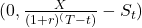Put Option Upper Bound=X
max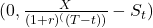< pt ≤ X

Example: Call Option Upper and Lower Bounds

(This is Example 4 from the curriculum.)

Consider a one-year call option with an exercise price, X, of EUR 1,000. The underlying asset, S0, trades at EUR 990 at time t = 0 and the risk-free rate, r, is 1%. What are the no-arbitrage upper and lower bounds in six months’ time if the underlying asset price, St, equals EUR 1,050?

Solution:

Call option lower bound=max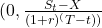Call option lower bound=max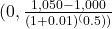= max⁡(0,54.96) = 54.96
Call option upper bound=St
Call option upper bound=1,050

## 7. Replication

Call Option Replication

To replicate a call option at contract inception (t=0), we must borrow at the risk-free rate, r, and use the proceeds to purchase the underlying at a price of S0.

At expiration, there are two possible replication outcomes:

• Exercise (ST > X): We should sell the underlying for ST and use the proceeds to repay X.
• No exercise (ST < X): No settlement is required.

If exercise were certain, we would borrow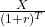at inception(as is the case of a forward). However, since exercise is uncertain, we borrow a proportion ofbased on the likelihood of exercise. This proportion depends on the moneyness of the option and the replicating transaction has to be adjusted as the likelihood of exercise changes. Therefore, as compared to replicating trades of a forward which remain constant, the replicating trades of an option are dynamic.

Put Option Replication

To replicate a put option at contract inception (t=0), we must sell the underlying short at a price of S0 and lend the proceeds at the risk-free rate, r.

At expiration, there are two possible replication outcomes:

• Exercise (ST < X): We should purchase the underlying for ST from the proceeds of the risk free loan.
• No exercise (ST > X): No settlement is required.

If exercise were certain, we would lendat inception(as is the case of a forward).
However, as is the case with call option, since exercise is uncertain, we lend a proportion of [latex]{X}(1+r)^T}[latex] based on the likelihood of exercise. The replicating transaction has to be adjusted as the likelihood of exercise changes.

## 8. Factors Affecting Option Value

Several factors affect the value of call and put options. We list these factors below:

Value of the Underlying

A call option becomes more valuable as the spot price of the underlying rises. Whereas, A put option becomes more valuable as the spot price of the underlying falls.

Relationship between the value of the option and the value of the underlying

• The value of a call option is directly related to the value of the underlying.
• The value of a put option is inversely related to the value of the underlying.
• The more ‘in-the-money’ an option is, the more sensitive the option’s value to the underlying price.

Exercise Price

Consider two call options with similar attributes (time to expiration, underlying) but different exercise prices. Assume the exercise price for call option 1 is 25 and that for call option 2 is 30. The right to buy at a lower price of 25 will be more valuable than the right to buy at a higher price of 30.

Similarly, in the case of a put option, the right to sell for a higher price is more valuable than the right to sell for a lower price.

Relationship between the value of the option and the exercise price

• The value of a European call option is inversely related to the exercise price.
• The value of a European put option is directly related to the exercise price.

Time to Expiration

Longer-term options should be worth more than shorter-term options. For instance, you have a bought a call option on a stock at an exercise price of \$25. Which one would be more valuable to you; an option that expires in a week or an option that expires a year later? Without doubt, the option that expires a year later as it gives enough time for the stock price to increase and make the option valuable.

But, is it true for a put option? Partly, yes. The more the time to expiration, the more the opportunity for the stock to fall below its exercise price. However, because put option buyers must wait for the underlying to be sold in order to receive (X – ST) upon exercise, the present value of the payoff decreases as the time to expiry increases. While less common, a longer time to expiration will reduce the value of a put in some cases, particularly for deep-in-the-money puts with a longer time to expiration and a higher risk-free rate of interest.

Relationship between the value of the option and the time to expiration

• The value of a call option is directly related to the time to expiration.
• The value of a put option can be directly or inversely related to the time to expiration. Inversely related under these conditions: the option is deep in the money, and the risk-free rate is high.

Risk-Free Interest Rate

As seen earlier, the exercise values of call and put options are computed as:

Call Option Exercise Value: Max (0, (St − PV(X)))

Put Option Exercise Value: Max (0, (PV(X) − St))

A higher risk-free rate lowers the PV(X). Therefore, a higher risk-free rate will increase the exercise value of a call option and decrease the exercise value of a put option.

Instructor’s Note: The risk-free rate does not directly affect the time value of an option.

Relationship between the value of the option and the risk-free interest rate

• The value of a call option is directly related to the risk-free interest rate.
• The value of a put option is inversely related to the risk-free interest rate.

Volatility of the Underlying

Volatility is good for both call and put options. If the underlying stock becomes more volatile, then the probability of the options expiring deep in the money becomes greater.

Relationship between the value of the option and the volatility of the underlying

• The value of a call option is directly related to the volatility of the underlying.
• The value of a put option is directly related to the volatility of the underlying.

Income or Cost Related to Owning Underlying Asset

 Call option Put option Benefits (dividends on stocks, interest on bonds, convenience yield on commodities) Stocks and bonds fall in value when dividends and interest are paid. Decreases because call holders do not get these benefits. Increases Carrying costs Increases as call holders do not incur these costs. Decreases

Relationship between the value of the option and benefits/costs of the underlying

• A call option is worth less the more benefits that are paid by the underlying. The call option is worth more the more the costs that are incurred in holding the underlying.
• A put option is worth more the more benefits that are paid by the underlying. The put option is worth less the more costs that are incurred in holding the underlying.

A summary of these factors is presented below.

 Increase in Value of call option will Value of put option will Value of the underlying Increase Decrease Exercise price Decrease Increase Risk-free rate Increase Decrease Time to expiration Increase Increase or Decrease Volatility of the underlying Increase Increase Costs incurred while holding the underlying Increase Decrease Benefits received while holding the underlying Decrease Increase

LIVE WEEKEND CLASSES *For 2024 Exam candidates*
This is default text for notification bar